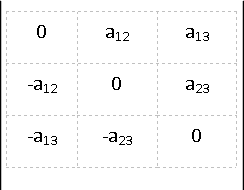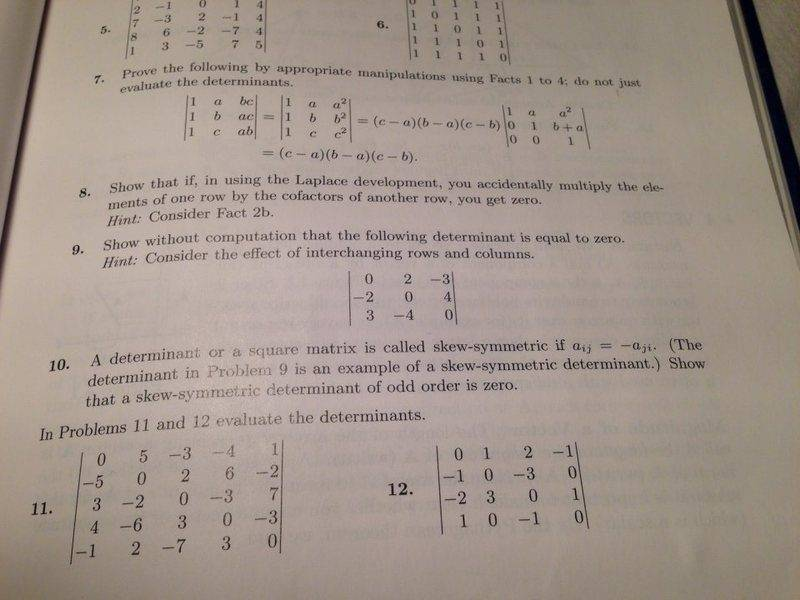# Prove 3x3 Skew symmetric matrix determinant is equal to zero

## Homework Statement

Hi there,

I'm happy with the proof that any odd ordered matrix's determinant is equal to zero. However, I am failing to see how it can be done specifically for a 3x3 matrix using only row and column interchanging.

## Homework Equations

I have attached the determinant as an image## The Attempt at a Solution

So my attempt was to try and collect a row of zeros via interchanging rows/columns. However it seems to be me that trying to move a zero into position by moving its row/column then affects the row/column containing another zero and so I can't ever get them into the same row.

Ray Vickson
Homework Helper
Dearly Missed

## Homework Statement

Hi there,

I'm happy with the proof that any odd ordered matrix's determinant is equal to zero. However, I am failing to see how it can be done specifically for a 3x3 matrix using only row and column interchanging.

## Homework Equations

I have attached the determinant as an image
View attachment 102053

## The Attempt at a Solution

So my attempt was to try and collect a row of zeros via interchanging rows/columns. However it seems to be me that trying to move a zero into position by moving its row/column then affects the row/column containing another zero and so I can't ever get them into the same row.

Write it out in detail, using
http://www.coolmath.com/algebra/14-determinants-cramers-rule/02-determinants-3x3-method-1-03

Why not just evaluate the determinant directly? Since it is specifically a 3 by 3 matrix?

But if you really want to do it using the invariant operations, you need an additional one for this example apart from basic row or column interchanging - the determinant doesn't change when you add a multiple of a column/row to another.

Thank you for your replies; the question stipulated not to find the determinant directly, and gave a hint concerning the interchanging of rows and columns; I presumed that it did not mean use addition of rows and columns. Fightfish, to clarify, are you saying it is impossible to complete using only row and column swaps?

I attach the question; it is number 9. I was wondering if it could be applied more generally.Mark44
Mentor
Call your matrix of problem 9 (in image) A. If you can apply row or column switches to get from |A| to -|AT, then the determinant is equal to 0.

In other words, if you can do this |A| = -|A1| = |A2| = ... -|AT|, then you're almost done. (Here the A1, A2, and so on are the different matrices you get from the row/column operations.) The reason is that a matrix and its transpose have the same determinant, so if |A| = k, a nonzero number, and -|AT| = k, then k has to be zero.

Now, as far as getting from |A| to |AT|, I have no idea which row/column switcheroos might produce this, but I believe that's the direction they're leading you.
"The details are left to the reader."

Do you have to use only row and column operations? If not, a simple way to do this would be to recognize that since ##A## is skew-symmetric then ##A = -A^t##, so ##\det(A) = \det(-A^t) = (-1)^3 \det(A^t) = -\det(A^t)##. Now show that the determinant of ##A^t## vanishes, which completes your proof.